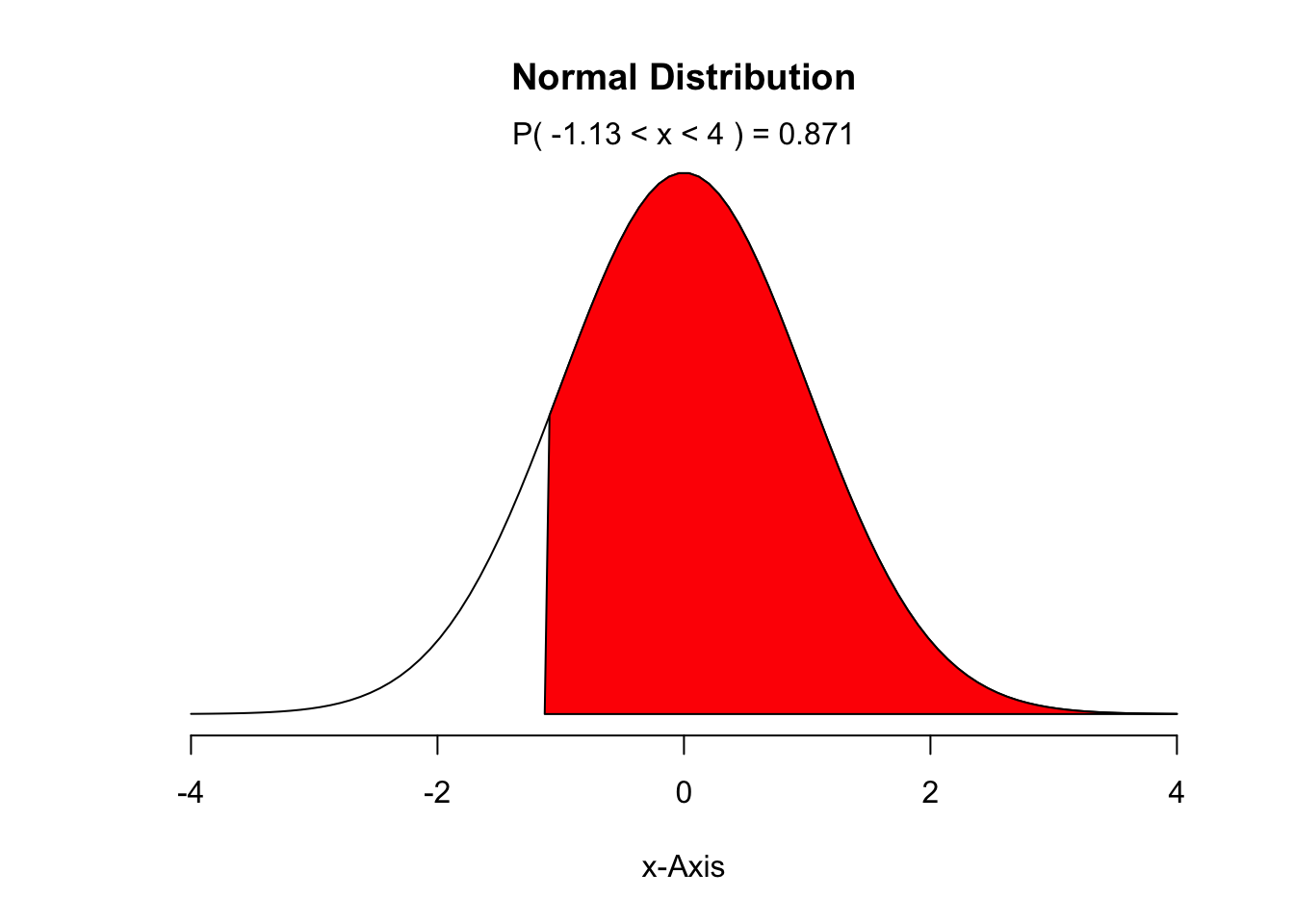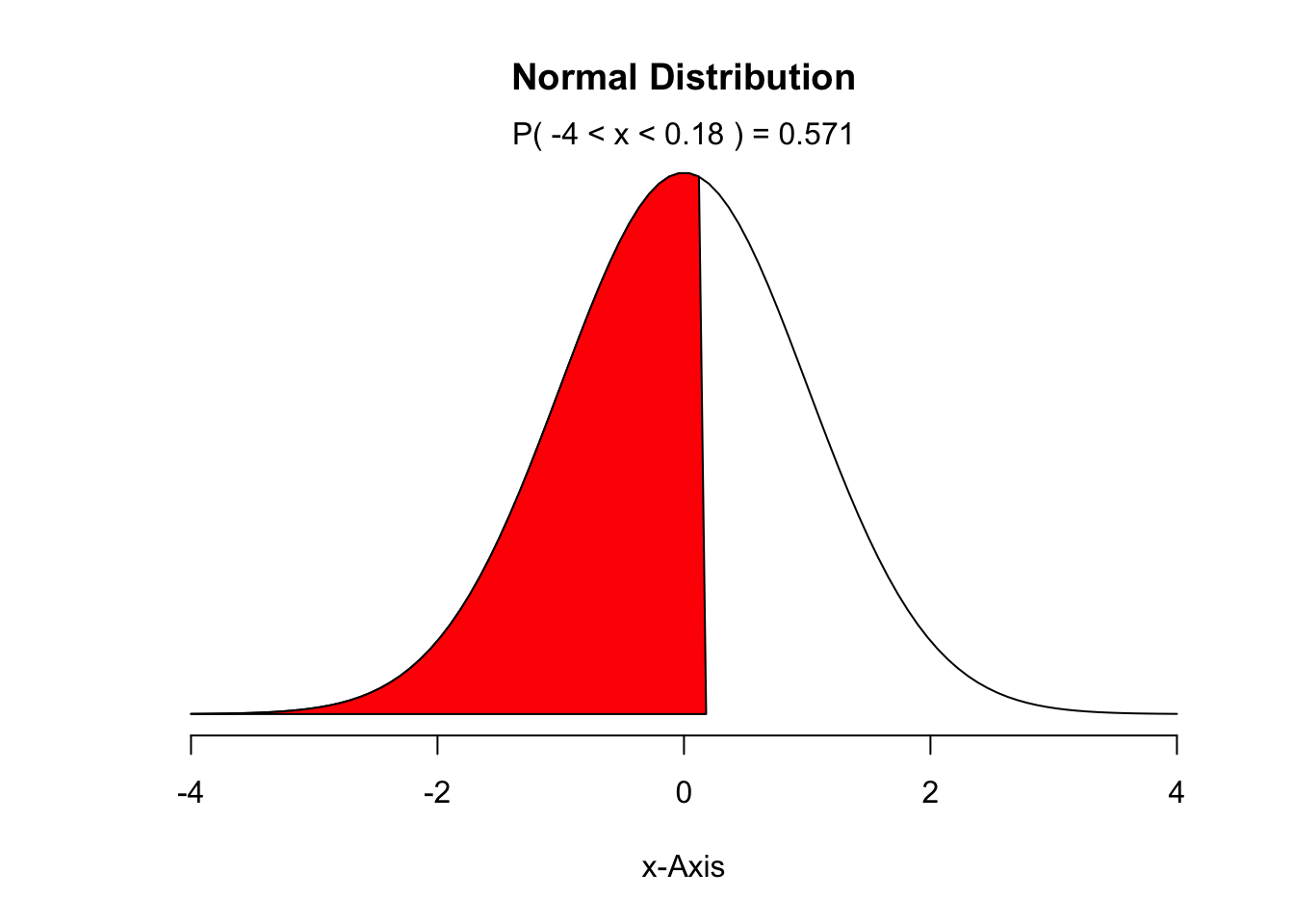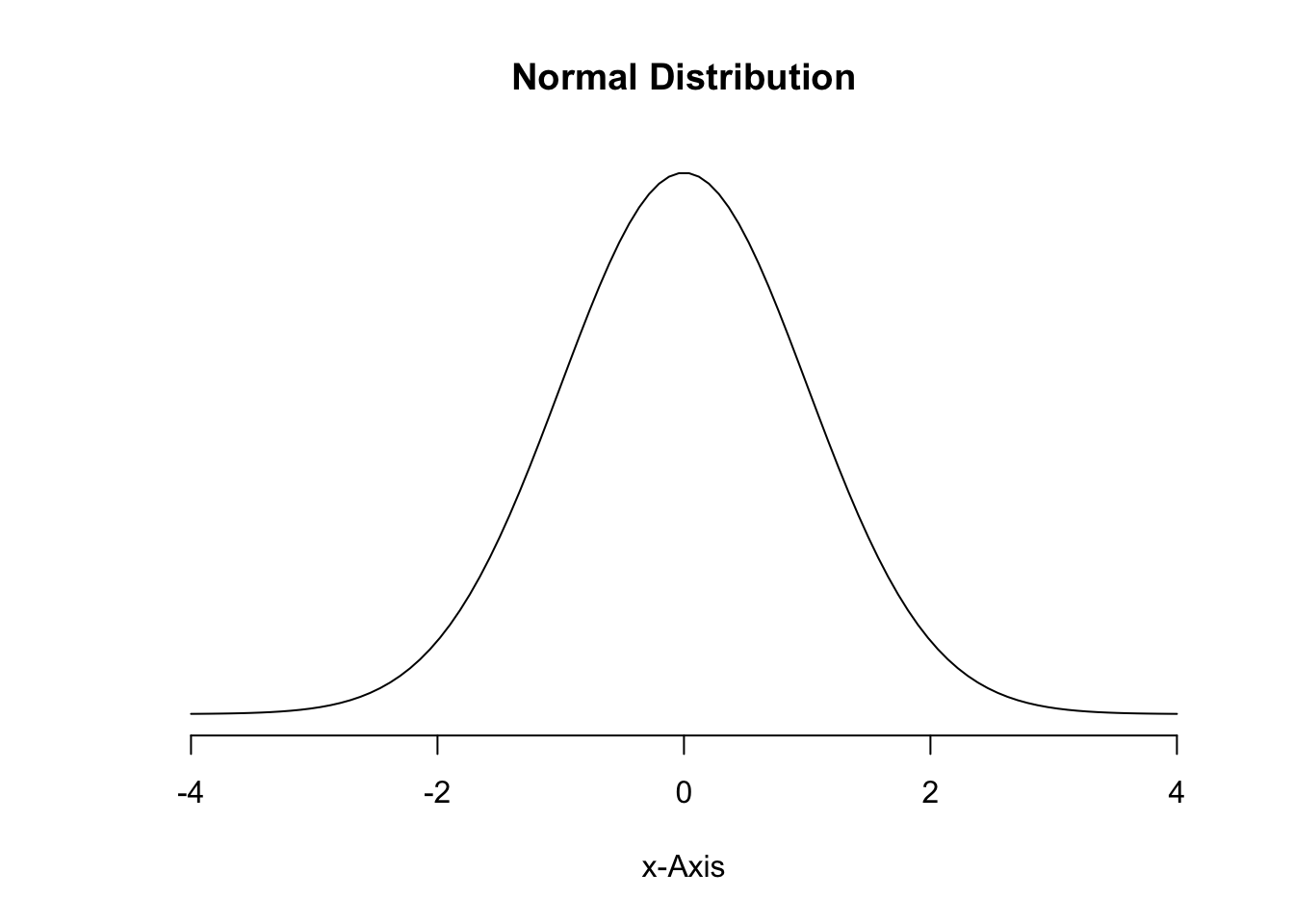#### Elina Azrilyan

##### 10/30/2018

Chapter 3 - Distributions of Random Variables Practice: 3.1 (see normalPlot), 3.3, 3.17 (use qqnormsim from lab 3), 3.21, 3.37, 3.41 Graded: 3.2 (see normalPlot), 3.4, 3.18 (use qqnormsim from lab 3), 3.22, 3.38, 3.42

3.2. What percent of a standard normal distribution N(μ = 0, sd = 1) is found in each region? Be sure to draw a graph.

1. Z >-1.13
``library(DATA606)``
``````##
## Welcome to CUNY DATA606 Statistics and Probability for Data Analytics
## This package is designed to support this course. The text book used
## is OpenIntro Statistics, 3rd Edition. You can read this by typing
## vignette('os3') or visit www.OpenIntro.org.
##
## The getLabs() function will return a list of the labs available.
##
## The demo(package='DATA606') will list the demos that are available.``````
``normalPlot(mean = 0, sd = 1, bounds = c(-1.13, 4))``1. Z <0.18
``normalPlot(mean = 0, sd = 1, bounds = c(-4, 0.18))``1. Z >8
``normalPlot(mean = 0, sd = 1, bounds = c(8, 8))``1. |Z|<0.5
``normalPlot(mean = 0, sd = 1, bounds = c(-4, -.5))``